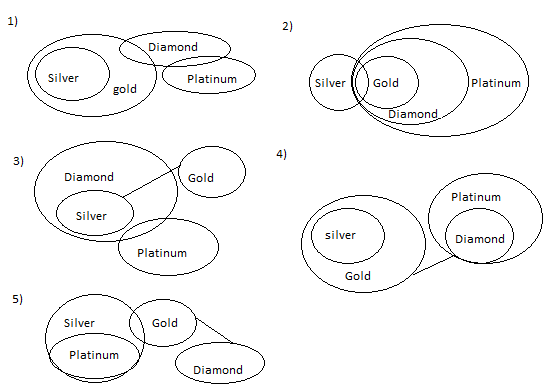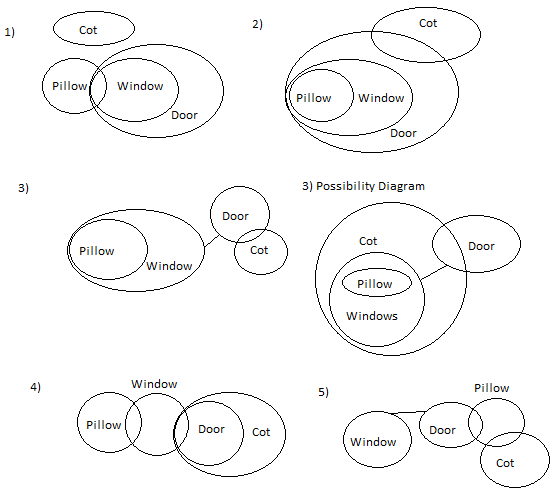# Reasoning Practice Quiz for SBI PO Mains

Reasoning Practice Quiz for SBI PO Mains
Directions (1 – 5): Each of the questions below consists of a question and two statements numbered I and II given below it. You have to decide whether the data provided in the statements are sufficient to answer the question. Read both the statements and give answer-
1. Who amongst Rahul, Ankit, Veena, Pooja and Vijay is the first to take lecture?
I. Rahul takes lecture before Pooja.
II. Veena is not the first to take lecture.
III. Pooja is not the last to take the lecture.
A) Only Statement I is sufficient to answer the question.
B) Only Statements I & II are sufficient to answer the question.
C) Only Statements I & either II or III sufficient to answer the question.
D) All three Statements are necessary to answer the question.
E) Question cannot be answered even with the information in all three statements.
2. Is Rekha the granddaughter of Seema?
I. The only sister of Reema is the mother of Rekha’s brother, Rahul.
II. Seema, the mother of Reema has only one grandson, Rahul.
A) Statement I alone is sufficient to answer the question, while statement II alone is not sufficient to answer the question.
B) Statement II alone is sufficient to answer the question, while statement I alone is not sufficient to answer the question.
C) Statement I alone or statement II alone is sufficient to answer the question.
D) Both the statements I and II are not sufficient to answer the question.
E) Both the statements I and II together are necessary to answer the question.
3. In which direction is point R with respect to P?
(i) C is in the north of P and R is in the west of C.
(ii) Q is in west of P and R is in the North of Q.
(iii) R is in the north-west direction of P.
A) Statement (i) and statement (ii) are sufficient to answer the question.
B) Statement (i) alone is sufficient to answer the question.
C) Either statement (i) alone or statement (ii) alone or statement (iii) alone is sufficient to answer the question.
D) All the statement (i), statement (ii) and statement (iii) are sufficient to answer the question.
E) None of the statement (i), statement (ii) and statement (iii) is sufficient to answer the question
4. How is X related to Z?
I. R is the cousin of P and the only son of X.
II. R is the only grandson of G, who has two children Y and Z.
III. P is the only son of Y, who is the only sister of her brother.
A) Only I and II
B) Only II and III
C) All I, II and III
D) Data inadequate
E) None of these
5. Among P, Q, R, S and T, each with a different weight, who is the lightest?
I. R is heavier than Q but lighter than T.
II. P is heavier than Q but lighter than S.
III. T is not the heaviest.
A) Only I and II
B) Only II and III
C) Only I and III
D) Data inadequate
E) None of these
Directions (6 – 8): In the following questions, only one Conclusion is given and five statements are given as 1), 2), 3), 4) and 5). From this you have to take the statements to be true even if they seem to be at variance with commonly known facts and then decide which of the given statement logically follows.
6. Conclusions:
I. Some silver are platinum.
II. Some diamonds are silver.
A) Statements I: All silver are gold. Some gold are diamond. No diamond is platinum.
B) Statements II: Some silvers are gold. All gold are diamond. All diamond is platinum.
C) Statements III: All silvers are diamond. No gold is silver. Some diamond is platinum.
D) Statements IV: All silvers are gold. No gold is diamond. All diamond is platinum
E) Statements V: All platinum are silver. Some gold is silver. No gold is diamond.
7. Conclusions:
I. Some white are not brown.
II. Some maroon are brown.
A) Statements I: All whites are black. All black are brown. No brown is maroon.
B) Statements II: Some white are black. All blacks are brown. All brown are maroon.
C) Statements III: Some black are white. No black is brown. No brown is maroon.
D) Statements IV: All white are black. No black is brown. All brown are maroon.
E) Statements V: No white is black. No black is brown. No brown is maroon.
8. Conclusions:
I. All windows being cot is a possibility.
II. Some doors are not pillow.
A) Statements I: Some pillows are window. All windows are door. No door is cot.
B) Statements II: All pillows are window. All windows are door. Some door is cot.
C) Statements III: All pillows are window. No window is door. Some door is cot.
D) Statements IV: Some pillows are window. Some window is door. All doors are cot.
E) Statements V: No window is door. Some door is pillow. Some pillow is cot
Directions (9 – 10): In the following questions, the symbols &, #, @, © and \$ are used with following meanings as illustrated below.
‘P & Q’ means ‘P is not greater than Q’.
‘P # Q’ means ‘P is not smaller than Q’.
‘P @ Q’ means ‘P is neither smaller than nor equal to Q’.
‘P © Q’ means ‘P is neither greater than nor equal to Q’.
‘P \$ Q’ means ‘P is neither greater than nor smaller than Q’.
In each question four statements showing relationship have been given, which are followed by four conclusions I, II, III and IV. Assuming that the given statements are true, find out which conclusions (s) is/are definitely true.
9. Statements: G # E, E \$ Q, Q @ R, H © R
Conclusions:
I. G \$ Q
II. Q © G
III. H © E
IV. R © G
A) Only either I or II is true
B) Only III is true
C) Only IV is true
D) III and IV are true
E) Only either I or II and III and IV are true
10. Statements: U @ S, S & Q, Q © T, T \$ N
Conclusions:
I. U @ Q
II. N @ S
III. T @ S
IV. N @ U
A) I and IV are true
B) II and III are true
C) I and III are true
D) II and IV are true
E) None of the above
Solutions:
1. E) By solving statement I: Rahul takes lecture before Pooja.
Rahul ¬ Pooja
II. Veena is not the first to take lecture.
III. Pooja is not the last to take the lecture.
Therefore, from three given statement we can’t conclude that who is first to take lecture.
Hence, question cannot be answered even with the information in all three statements.
2. E)   3. C)   4. C)   5. A)
6. B)Therefore as we can see in above diagram only statement of option 2) represent given conclusion.
7. D)Therefore as we can see in above diagram only statement of option 4) represent given conclusion.
8. C)Therefore as we can see in above diagram only statement of option 3) represent given conclusion.
(9 – 10):
From the given conditions, we draw the following table:
 P is & # @ © \$ ≤ ≥ > < = Than Q
9. E) Given statements are: G # E, E \$ Q, Q @ R, H © R
On replacing symbols: G ≥ E, E = Q, Q > R, H < R
On combining: G ≥ E = Q > R > H
Conclusions:
I. G \$ Q G = Q (False as G Q)
II. Q © G Q < G (False as G ≥ Q)
III. H © E H < E (Clearly, True)
IV. R © G R < G (Clearly, True)
Thus only conclusion III & IV is true but conclusion I and II form a complementary pair.
Hence either I or II and III & IV is true.
10. B) Given statements are: U @ S, S & Q, Q © T, T \$ N
On replacing symbols: U > S, S ≤ Q, Q < T, T = N
On combining: U > S ≤ Q < T = N
Conclusions:
I. U @ Q U > Q (False as clear relation between U & Q cant be established)
II. N @ S N > S (Clearly, True)
III. T @ S T > S (Clearly, True)
IV. N @ U N > U (False as clear relation between N & U cant be established)
Thus only conclusion II and III are true.